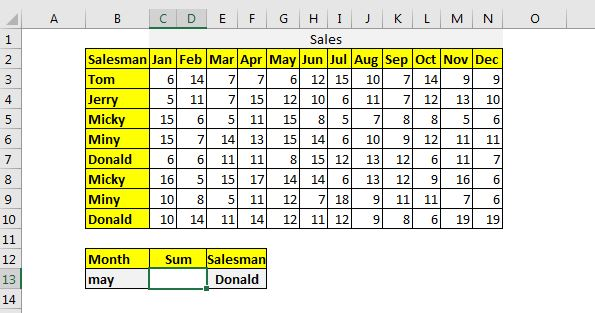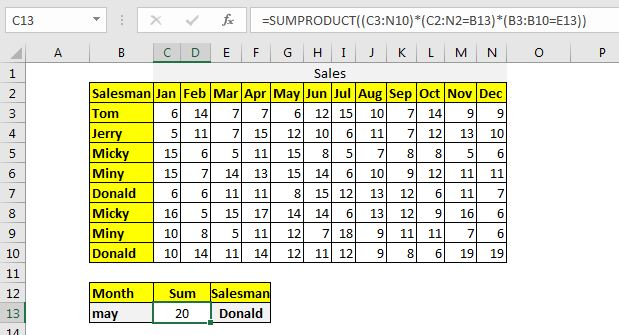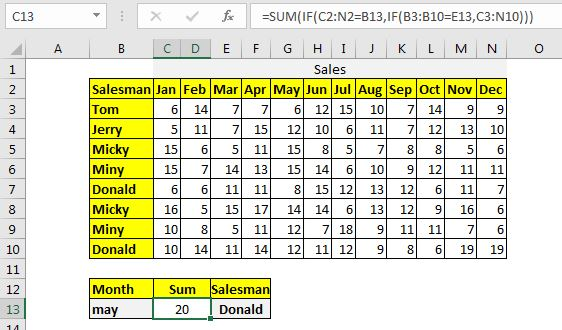# How to Sum by Matching Row and Column in Excel

So far we have learned how to sum the entire matching column of a table in Excel. But how do we sum values when we need to match column and row. In this article, we will learn how to do the sum of matching rows and columns.

There are two formulas to do this but first, let us the scenario.

Here, I have a table that records the sales done by employees in different months. The name of employees can repeat. See the below figure:We need to get the sum of the May month where the salesman is Donald.

### Method 1: Summing up the matching column header and row header Using the SUMPRODUCT function.

The SUMPRODUCT Function is the most versatile function when it comes to sum and count values with tricky criteria. The generic function to sum by matching column and row is:

Columns: It is the 2-dimensional range of the columns that you want to sum. It should not contain headers. In the table above it is C3:N7.

column_headers: It is the header range of columns that you want to sum. In the above data, it is C2:N2.

column_headingIt is the heading that you want to match. In the example above, it is in B13.

Without further delay let's use the formula.

row_headers: It is the header range of rows that you want to sum. In the above data, it is B3:B10.

row_headingIt is the heading that you want to match in rows. In the example above, it is in F13 .

Without further delay let's use the formula.

Write this formula in cell D13 and let the excel do it's magic (there's no such thing as magic)...

 =SUMPRODUCT((C3:N10)*(C2:N2=B13)*(B3:B10=E13))

This returns the value:Now when you change the month or salesman the sum will change as per the row heading and column heading.

How does it work?

This simple boolean logic.

(C2:N2=B13): This statement will return an array of TRUE and FALSE. All the matching values in the column have true and others will have false. In this case, we will have only one True as the range C2:N2 only contains one instance May at 5th Location.

(B3:B10=E13): This will work the same as above and will return an array TRUE and FALSE. All the matching values will have TRUE and others will have FALSE. In this case, we will have 2 TRUEs as the range B3:B10 has two instances of "Donald".

(C2:N2=B13)*(B3:B10=E13): Now we are multiplying the arrays returned by statements. This will implement and logic and we will get an array of 1s and 0s. Now we will have a 2D array that will contain 2 1s and rest 0s.

(C3:N10)*(C2:N2=B13)*(B3:B10=E13)= Finally we multiply the 2D array with the 2D table. It will return again an array of 0s and the numbers that match the criteria.

Finally, the SUMPRODUCT function will sum up the array which will result in the desired output.

### Method 2: Summing up the matching column header and row header Using the SUM and IF function

The generic formula for summing matching row and column using SUM and IF Excel function is:

All the variables are the same as in the above-explained method. Here, they just need to be used in a different order.

Write this formula in cell D13:

 =SUM(IF(C2:N2=B13,IF(B3:B10=E13,C3:N10)))

This returns the correct answer. See the screenshot below:How does it work?

The logic is the same as the first SUMPRODCUT method only the mechanism is different. If I explain it in short, the inner IF Function returns a 2D array of the same dimension as the table. This array contains the number of two matched rows. Then the inner IF Function matches the two-column headings in this array and returns the 2D array that only contains the numbers that match both, column and heading. All other elements of the array will be FALSE.

Finally, the SUM function sums up this array and we get our sum.

So yeah guys, this how you can sum up the matching rows and columns from a table in excel. I hope it was explanatory and useful to you. If you have any doubts regarding this topic or have any other excel/VBA related doubt, ask in the comments section below.

Related Articles:

How to Sum Column in a Excel by Matching Heading | If you want to get the sum of a column by just using the column name, you can do this in 3 easy ways in Excel. The syntax of the SUMPRODUCT method to sum matching column is:

SUMIF with 3D Reference in Excel | The fun fact is that the normal Excel 3D referencing does not work with conditional functions, like SUMIF function. In this article, we will learn how to get 3D referencing working with SUMIF function.

Relative and Absolute Reference in Excel | Referencing in excel is an important topic for every beginner. Even experienced excel users do mistakes in referencing.

Dynamic Worksheet Reference | Give reference sheets dynamically using the INDIRECT function of excel. This is simple...

Expanding References in Excel | The expanding reference expands when copied down or rightwards. We use the \$ sign before the column and row number to do so. Here is one example...

All About Absolute Reference | The default reference type in excel is relative but if you want the reference of cells and ranges to be absolute use the \$ sign. Here are all the aspects of absolute referencing in Excel.

Popular Articles:

50 Excel Shortcuts to Increase Your Productivity | Get faster at your task. These 50 shortcuts will make your work even faster on Excel.

The VLOOKUP Function in Excel | This is one of the most used and popular functions of excel that is used to lookup value from different ranges and sheets.

COUNTIF in Excel 2016 | Count values with conditions using this amazing function. You don't need filter your data to count specific value. Countif function is essential to prepare your dashboard.

How to Use SUMIF Function in Excel | This is another dashboard essential function. This helps you sum up values on specific conditions.

Terms and Conditions of use

The applications/code on this site are distributed as is and without warranties or liability. In no event shall the owner of the copyrights, or the authors of the applications/code be liable for any loss of profit, any problems or any damage resulting from the use or evaluation of the applications/code.